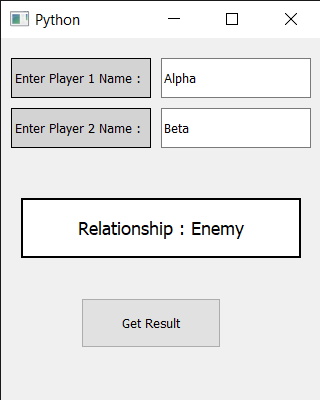# PyQt5 – Flames Calculator

• Last Updated : 03 Jun, 2022

In this article we will see how we can create a flames calculator using PyQt5. This flames calculator assesses and predicts the outcome of a relationship based on an algorithm of two given names.
FLAMES is a popular game named after the acronym: Friends, Lovers, Affectionate, Marriage, Enemies, Sibling. This game does not accurately predict whether or not an individual is right for you, but it can be fun to play this with your friends.
Below is how the flames calculator will look likeGUI implementation steps :
1. Create a label that says enter player 1 name and set color and geometry to it
2. Add QLineEdit widget in front of the first name label to get the first name
3. Similarly create another label that says enter player 2 name and set color and geometry to it
4. Add QLineEdit widget in front of this label to get the second name
5. Create a label to show the result and set its border, geometry and change its font.
6. Create a push button at the bottom which says get result.
Back end implementation steps :
1. Add action to the push button
2. Inside the push button action get both player names
3. Remove the spacing in between the names
4. Call the get result method that returns the result
5. Inside the get result method call the remove letter method that removes the common characters with their respective common occurrences.
6. Then get the count of characters that are left and take FLAMES letters as [“F”, “L”, “A”, “M”, “E”, “S”]
7. Start removing letter using the count we got. The letter which last the process is the result, return the result
8. Set the result to the label using setText method.

Below is the implementation

## Python3

 `# importing libraries``from` `PyQt5.QtWidgets ``import` `*``from` `PyQt5 ``import` `QtCore, QtGui``from` `PyQt5.QtGui ``import` `*``from` `PyQt5.QtCore ``import` `*``import` `sys`  `class` `Window(QMainWindow):` `    ``def` `__init__(``self``):``        ``super``().__init__()` `        ``# setting title``        ``self``.setWindowTitle(``"Python "``)` `        ``# setting geometry``        ``self``.setGeometry(``100``, ``100``, ``320``, ``400``)` `        ``# calling method``        ``self``.UiComponents()` `        ``# showing all the widgets``        ``self``.show()` `    ``# method for components``    ``def` `UiComponents(``self``):` `        ``# creating label to tell user enter first name``        ``name1_label ``=` `QLabel(``"Enter Player 1 Name : "``, ``self``)` `        ``# setting border and color to the label``        ``name1_label.setStyleSheet("border : ``1px` `solid black ;``                                    ``background : lightgrey;")` `        ``# setting geometry``        ``name1_label.setGeometry(``10``, ``20``, ``140``, ``40``)` `        ``# creating label to tell user enter second name``        ``name2_label ``=` `QLabel(``"Enter Player 2 Name : "``, ``self``)` `        ``# setting border and color to the label``        ``name2_label.setStyleSheet("border : ``1px` `solid black ;``                                     ``background : lightgrey;")` `        ``# setting geometry``        ``name2_label.setGeometry(``10``, ``70``, ``140``, ``40``)` `        ``# creating a line edit to get the first name``        ``self``.name1 ``=` `QLineEdit(``self``)` `        ``# setting geometry``        ``self``.name1.setGeometry(``160``, ``20``, ``150``, ``40``)` `        ``# creating a line edit to get the second name``        ``self``.name2 ``=` `QLineEdit(``self``)` `        ``# setting geometry``        ``self``.name2.setGeometry(``160``, ``70``, ``150``, ``40``)` `        ``# creating a label to show result``        ``self``.output ``=` `QLabel(``"Find Relationship Status"``, ``self``)` `        ``# setting geometry to the output label``        ``self``.output.setGeometry(``20``, ``160``, ``280``, ``60``)` `        ``# setting border and background color to it``        ``self``.output.setStyleSheet("border : ``2px` `solid black;``                                       ``background : white;")` `        ``# setting alignment to output``        ``self``.output.setAlignment(Qt.AlignCenter)` `        ``# setting font to the output``        ``self``.output.setFont(QFont(``'Times'``, ``11``))` `        ``# creating push button to get result``        ``self``.push ``=` `QPushButton(``"Get Result"``, ``self``)` `        ``# setting geometry tot he button``        ``self``.push.setGeometry(``80``, ``260``, ``140``, ``50``)` `        ``# adding action to the push button``        ``self``.push.clicked.connect(``self``.do_action)` `    ``# action called by the push button``    ``def` `do_action(``self``):` `        ``# getting names``        ``name1 ``=` `self``.name1.text()``        ``name2 ``=` `self``.name2.text()` `        ``# removing spacing form the name``        ``name1.replace(``" "``, "")``        ``name2.replace(``" "``, "")`  `        ``# function for removing common characters``        ``# with their respective occurrences``        ``def` `remove_match_char(list1, list2):` `            ``for` `i ``in` `range``(``len``(list1)):``                ``for` `j ``in` `range``(``len``(list2)):` `                    ``# if common character is found``                    ``# then remove that character``                    ``# and return list of concatenated``                    ``# list with True Flag``                    ``if` `list1[i] ``=``=` `list2[j]:``                        ``c ``=` `list1[i]` `                        ``# remove character from the list``                        ``list1.remove(c)``                        ``list2.remove(c)` `                        ``# concatenation of two list elements with *``                        ``# * is act as border mark here``                        ``list3 ``=` `list1 ``+` `[``"*"``] ``+` `list2` `                        ``# return the concatenated list with True flag``                        ``return` `[list3, ``True``]` `                        ``# no common characters is found``            ``# return the concatenated list with False flag``            ``list3 ``=` `list1 ``+` `[``"*"``] ``+` `list2``            ``return` `[list3, ``False``]` `        ``# method to find the result``        ``def` `find_relation(p1_list, p2_list):``            ` `            ``# taking a flag as True initially``            ``proceed ``=` `True` `            ``# keep calling remove_match_char function``            ``# until common characters is found or``            ``# keep looping until proceed flag is True``            ``while` `proceed:``                ``# function calling and store return value``                ``ret_list ``=` `remove_match_char(p1_list, p2_list)` `                ``# take out concatenated list from return list``                ``con_list ``=` `ret_list[``0``]` `                ``# take out flag value from return list``                ``proceed ``=` `ret_list[``1``]` `                ``# find the index of "*" / border mark``                ``star_index ``=` `con_list.index(``"*"``)` `                ``# list slicing perform` `                ``# all characters before * store in p1_list``                ``p1_list ``=` `con_list[: star_index]` `                ``# all characters after * store in p2_list``                ``p2_list ``=` `con_list[star_index ``+` `1``:]` `                ``# count total remaining characters``            ``count ``=` `len``(p1_list) ``+` `len``(p2_list)` `            ``# list of FLAMES acronym``            ``result ``=` `[``"Friends"``, ``"Love"``, ``"Affection"``, ``"Marriage"``, ``"Enemy"``, ``"Siblings"``]` `            ``# keep looping until only one item``            ``# is not remaining in the result list``            ``while` `len``(result) > ``1``:` `                ``# store that index value from``                ``# where we have to perform slicing.``                ``split_index ``=` `(count ``%` `len``(result) ``-` `1``)` `                ``# this steps is done for performing``                ``# anticlock-wise circular fashion counting.``                ``if` `split_index >``=` `0``:` `                    ``# list slicing``                    ``right ``=` `result[split_index ``+` `1``:]``                    ``left ``=` `result[: split_index]` `                    ``# list concatenation``                    ``result ``=` `right ``+` `left` `                ``else``:``                    ``result ``=` `result[: ``len``(result) ``-` `1``]` `            ``# print final result``            ``return` `result[``0``]` `        ``# calling find relation method``        ``result ``=` `find_relation(``list``(name1), ``list``(name2))` `        ``# setting text to the output label``        ``self``.output.setText(``"Relationship : "` `+` `result)`  `# create pyqt5 app``App ``=` `QApplication(sys.argv)` `# create the instance of our Window``window ``=` `Window()` `# start the app``sys.exit(App.``exec``())`

Output :

My Personal Notes arrow_drop_up